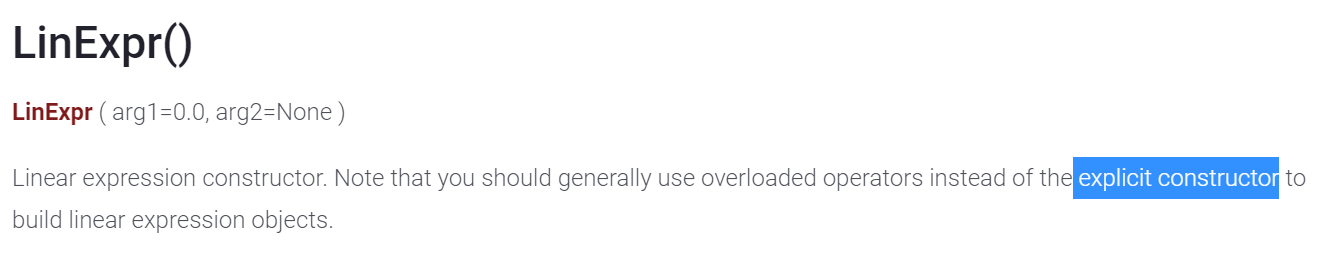•Gurobi Staff

Hi Trista,

You can check your thesis by writing a small script and checking the times

import gurobipy as gpfrom gurobipy import GRBimport numpy as npimport timeI = 1000J = 1000P = np.random.rand(I)C = np.random.rand(I,J)m = gp.Model()x = m.addVars(I)y = m.addVars(I,J)start = time.time()m.setObjective(    gp.quicksum(P[i] * x[i] for i in range(I)) -    gp.quicksum(C[i, j] * y[i, j] for i in range(I) for j in range(J))   )time_needed = time.time() - startprint("Time needed for 1st version: %f"%(time_needed))start = time.time()m.setObjective(    gp.quicksum(        P[i] * x[i] - gp.quicksum(            C[i, j] * y[i, j]            for j in range(J)        )        for i in range(I)    ))time_needed = time.time() - startprint("Time needed for 2nd version: %f"%(time_needed))

You will notice that there is no much difference between the two versions. However, you can significantly improve model building speed with the following version

start = time.time()lexpr = gp.LinExpr(0)for i in range(I):    lexpr.add(x[i], P[i])    for j in range(J):        lexpr.add(y[i,j], -C[i,j])m.setObjective(lexpr)time_needed = time.time() - startprint("Time needed for 3rd version: %f"%(time_needed))

You can read more on efficient model building in How do I improve the time to build my model?

Would the advantages keep consistant even in constraints?

Yes, if the constraints are build in a similar way.

May I assign the quicksum value to a variable and use the variable in both addConstrs and setObjectives?

Yes. The quicksum function returns an expression object which can be reused in other expression objects.

Note that Gurobi does not support strict inequalities. Thus

model.addConstr(tmp_var < 5)

is not available in Gurobi, only $$\leq$$. Moreover, it should be

model.setObjective(tmp_var, GRB.MAXIMIZE)

Best regards,
Jaromił

•Hello Jaromił,

Thanks for your promt reply. I look into the links you provided and did some search concerning LinExpr, quicksum, tupledict.sum, add, addTerms.

I tried different writing ways to solve the problem in network.py. Accoring to the same instance in it, I derive some observations. addConstr with for loop is faster than addConstrs. quicksum with for loop is faster than that with tupledict.select or tupledict.sum. I doubt that these observations are general enough and prove some universal prospositions.

However, I encountered that LinExpr may not help in effiency in this instance. I wonder what is the factor to make LinExpr dominant. Is it the nested structure of the expression to bring the advantage? Besides, one math equation with some summation may be written in LinExpr with add, addTerms, quicksum or +. Which one would be the fastest? In another case, if the right-hand-side equation is only a product of two objects, is it still faster to use LinExpr instead of * or tupledict.prod ? Is it more ideal to construct a expression with equality or inequality sign to form a constraint or make the LHS and RHS respectively and use them in addConstr?

I truely appreciate your kind response.

Best regards,

Trista

•Gurobi Staff

Hi Trista,

Gurobi methods should be more efficient than the methods built into the data structures. Thus, it makes sense that quicksum is faster than .select or .sum. It is also true that addConstr in a for-loop can be slightly faster than addConstrs in some cases.

Using LinExpr with addTerms should be fastest followed by add. However, there quicksum can outperform those in some cases. In general, the difference in speed  between the 3 should not be too big. However, the difference in speed is significant if you would construct your linear expressions via the += and *= operators.

In another case, if the right-hand-side equation is only a product of two objects, is it still faster to use LinExpr instead of * or tupledict.prod ?

If the number of objects is small, then there should be no significant difference.

Is it more ideal to construct a expression with equality or inequality sign to form a constraint or make the LHS and RHS respectively and use them in addConstr?

There should be no significant difference between those 2 options. I would recommend using the version which you find to be easier to read/understand.

In general, if model construction speed is crucial for your application, I would test different alternatives to find the best performing one for your particular model.

Best regards,
Jaromił

•Hi

Thanks for your promt reply. I have another relative question. The screenshot below is captured from the official website document.Could you please provide some examples of explicit constructors? I truely appreciate your time and help.

Best regards,

Trista

•Gurobi Staff

Hi Trista,

What the documentation means is

For linear expressions of moderate size (only a few terms) and for the ease of usage, you should generally use overloaded operators instead of the explicit constructor to build linear expression objects.

An example of an explicit constructor usage would be

X = []coeffs = []for i in range(1,1001):  X.append(m.addVar( name="X%d"%(i)))  coeffs.append(i)expr = gp.LinExpr(coeffs, X) # LinExpr constructor taking 2 listsm.addConstr(expr <= 5)

Best regards,
Jaromił

•I was trying to imitate this snipped in my code

lexpr = gp.LinExpr(0)for i in range(I):    lexpr.add(x[i], P[i])    for j in range(J):        lexpr.add(y[i,j], -C[i,j])
m.setObjective(lexpr)

y = m.addVars(frequencies, vtype=GRB.BINARY, name="y") #frequency "i" purchased {1 = yes, 0 = no }
x = m.addVars(frequencies,cities, vtype=GRB.BINARY, name="x") # frequency "i" is used in city "J" {1 = used, 0 = not used}

lexpr = gp.LinExpr(0)
for i in frequencies:
for j in cities:
TypeError                                 Traceback (most recent call last)~\AppData\Local\Temp/ipykernel_23848/3267894265.py in <module>      5     lexpr.add(y[i],-500000)      6     for j in cities:----> 7         lexpr.add(c[i],x[i,j])      8       9 m.setObjective(lexpr,GRB.MAXIMIZE)src\gurobipy\linexpr.pxi in gurobipy.LinExpr.add()src\gurobipy\linexpr.pxi in gurobipy.LinExpr.addConstant()TypeError: float() argument must be a string or a number, not 'gurobipy.LinExpr'